## Thursday, October 27, 2011

### All your Bayes are belong to us!

This week's post contains solutions to My Favorite Bayes's Theorem Problems, and one new problem.  If you missed last week's post, go back and read the problems before you read the solutions!

If you don't understand the title of this post, brush up on your memes.

1) The first one is a warm-up problem.  I got it from Wikipedia (but it's no longer there):
Suppose there are two full bowls of cookies. Bowl #1 has 10 chocolate chip and 30 plain cookies, while bowl #2 has 20 of each. Our friend Fred picks a bowl at random, and then picks a cookie at random. We may assume there is no reason to believe Fred treats one bowl differently from another, likewise for the cookies. The cookie turns out to be a plain one. How probable is it that Fred picked it out of Bowl #1?
First the hypotheses:
A: the cookie came from Bowl #1
B: the cookie came from Bowl #2

And the priors:
P(A) = P(B) = 1/2

The evidence:
E: the cookie is plain

And the likelihoods:
P(E|A) = prob of a plain cookie from Bowl #1 = 3/4
P(E|B) = prob of a plain cookie from Bowl #2 = 1/2

Plug in Bayes's theorem and get
P(A|E) = 3/5

You might notice that when the priors are equal they drop out of the BT equation, so you can often skip a step.

2) This one is also an urn problem, but a little trickier.
The blue M&M was introduced in 1995.  Before then, the color mix in a bag of plain M&Ms was (30% Brown, 20% Yellow, 20% Red, 10% Green, 10% Orange, 10% Tan).  Afterward it was (24% Blue , 20% Green, 16% Orange, 14% Yellow, 13% Red, 13% Brown).
A friend of mine has two bags of M&Ms, and he tells me that one is from 1994 and one from 1996.  He won't tell me which is which, but he gives me one M&M from each bag.  One is yellow and one is green.  What is the probability that the yellow M&M came from the 1994 bag?
Hypotheses:
A: Bag #1 from 1994 and Bag #2 from 1996
B: Bag #2 from 1994 and Bag #1 from 1996

Again, P(A) = P(B) = 1/2.

The evidence is:
E: yellow from Bag #1, green from Bag #2

We get the likelihoods by multiplying the probabilities for the two M&M:

P(E|A) = (0.2)(0.2)
P(E|B) = (0.1)(0.14)

For example, P(E|B) is the probability of a yellow M&M in 1996 (0.14) times the probability of a green M&M in 1994 (0.1).

Plugging the likelihoods and the priors into Bayes's theorem, we get P(A|E) = 40 / 54 ~ 0.74

By introducing the terms Bag #1 and Bag #2, rather than "the bag the yellow M&M came from" and "the bag the green came from," I avoided the part of this problem that can be tricky: keeping the hypotheses and the evidence straight.

3) This one is from one of my favorite books, David MacKay's Information Theory, Inference, and Learning Algorithms:
Elvis Presley had a twin brother who died at birth.  What is the probability that Elvis was an identical twin?
To answer this one, you need some background information: According to the Wikipedia article on twins:  ``Twins are estimated to be approximately 1.9% of the world population, with monozygotic twins making up 0.2% of the total---and 8% of all twins.''

There are several ways to set up this problem; I think the easiest is to think about twin birth events, rather than individual twins, and to take the fact that Elvis was a twin as background information.

So the hypotheses are
A: Elvis's birth event was an identical birth event
B: Elvis's birth event was a fraternal twin event

If identical twins are 8% of all twins, then identical birth events are 8% of all twin birth events, so the priors are

P(A) = 8%
P(B) = 92%

The relevant evidence is
E: Elvis's twin was male

So the likelihoods are
P(E|A) = 1
P(E|B) = 1/2

Because identical twins are necessarily the same sex, but fraternal twins are equally likely to be opposite sex (or, at least, I assume so).  So

P(A|E) = 8/54 ~ 0.15.

The tricky part of this one is realizing that the sex of the twin provides relevant information!

4) Also from MacKay's book:
Two people have left traces of their own blood at the scene of a crime.  A suspect, Oliver, is tested and found to have type O blood.  The blood groups of the two traces are found to be of type O (a common type in the local population, having frequency 60%) and of type AB (a rare type, with frequency 1%).  Do these data (the blood types found at the scene) give evidence in favour [sic] of the proposition that Oliver was one of the two people whose blood was found at the scene?
For this problem, we are not asked for a posterior probability; rather we are asked whether the evidence is incriminating.  This depends on the likelihood ratio, but not the priors.

The hypotheses are
X: Oliver is one of the people whose blood was found
Y: Oliver is not one of the people whose blood was found

The evidence is
E: two blood samples, one O and one AB

We don't need priors, so we'll jump to the likelihoods.  If X is true, then Oliver accounts for the O blood, so we just have to account for the AB sample:

P(E|X) = 0.01

If Y is true, then we assume the two samples are drawn from the general population at random.  The chance of getting one O and one AB is

P(E|Y) = 2(0.6)(0.01) = 0.012

Notice that there is a factor of two here because there are two permutations that yield E.

So the evidence is slightly more likely under Y, which means that it is actually exculpatory!  This problem is a nice reminder that evidence that is consistent with a hypothesis does not necessarily support the hypothesis.

5) I like this problem because it doesn't provide all of the information.  You have to figure out what information is needed and go find it.
According to the CDC, ``Compared to nonsmokers, men who smoke are about 23 times more likely to develop lung cancer and women who smoke are about 13 times more likely.''
If you learn that a woman has been diagnosed with lung cancer, and you know nothing else about her, what is the probability that she is a smoker?
I find it helpful to draw a tree:

If y is the fraction of women who smoke, and x is the fraction of nonsmokers who get lung cancer, the number of smokers who get cancer is proportional to 13xy, and the number of nonsmokers who get lung cancer is proportional to x(1-y).

Of all women who get lung cancer, the fraction who smoke is 13xy / (13xy + x(1-y)).

The x's cancel, so it turns out that we don't actually need to know the absolute risk of lung cancer, just the relative risk.  But we do need to know y, the fraction of women who smoke.  According to the CDC, y was 17.9% in 2009.  So we just have to compute

13y / (13y + 1-y) ~ 74%

This is higher than many people guess.

6) Next, a mandatory Monty Hall Problem.  First, here's the general description of the scenario, from Wikipedia:
Suppose you're on a game show, and you're given the choice of three doors: Behind one door is a car; behind the others, goats. You pick a door, say Door A [but the door is not opened], and the host, who knows what's behind the doors, opens Door B, which has a goat. He then says to you, "Do you want to pick Door C?" Is it to your advantage to switch your choice?
The answer depends on the behavior of the host when the car is behind Door A.  In this case the host can open either B or C.  Suppose he chooses B with probability p and C otherwise.  What is the probability that the car is behind Door A (as a function of p)?

The hypotheses are
A: the car is behind Door A
B: the car is behind Door B
C: the car is behind Door C

And the priors are
P(A) = P(B) = P(C) = 1/3

The likelihoods are
P(E|A) = p, because in this case Monty has a choice and chooses B with probability p,
P(E|B) = 0, because if the car were behind B, Monty would not have opened B, and
P(E|C) = 1, because in this case Monty has no choice.

Applying Bayes's Theorem,
P(A|E) = p / (1+p)

In the canonical scenario, p=1/2, so P(A|E) = 1/3, which is the canonical solution.  If p=0, P(A|E) = 0, so you can switch and win every time (when Monty opens B, that it).  If p=1, P(A|E) = 1/2, so in that case it doesn't matter whether you stick or switch.

When Monty opens C, P(A|E) = (1-p) / (2-p)

[Correction: the answer in this case is not (1-p) / (1+p), which what I wrote in a previous version of this article.  Sorry!].

7) And finally, here is a new problem I just came up with:
If you meet a man with (naturally) red hair, what is the probability that neither of his parents has red hair?
Hints: About 2% of the world population has red hair.  You can assume that the alleles for red hair are purely recessive.  Also, you can assume that the Red Hair Extinction theory is false, so you can apply the Hardy–Weinberg principle.

Solution to this one next week!

Please let me know if you have suggestions for more problems. An ideal problem should meet at least some of these criteria:
1) It should be based on a context that is realistic or at least interesting, and not too contrived.
2) It should make good use of Bayes's Theorem -- that is, it should be easier to solve with BT than without.
3) It should involve some real data, which the solver might have to find.
4) It might involve a trick, but should not be artificially hard.

If you send me something that is not under copyright, or is usable under fair use, I will include it in the next edition of Think Stats and add you to the contributors list.

1.I don't understand the 3/5 answer to problem 1.

2.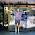By Bayes's Theorem, we have

P(A|E) = P(A) P(E|A) / P(E)

and

P(E) = P(A) P(E|A) + P(B) P(E|B)

So

1/2 3/4
-----------------
1/2 3/4 + 1/2 1/2

= 3/5.

Since the priors are equal, they drop out. So we could have skipped a step and just used the likelihoods.

3.In 3), I'm not sure how you came up with the statement "If identical twins are 8% of all twins, then identical birth events are 8% of all birth events", as the source you quote states that identical twin births are 0.2% of total births.

Yes, each member of a fraternal twin birth has an equal chance of being either sex (not opposite sex'd).

However, since the background of the problem states that Elvis had a twin brother who died at birth, the chance of that arrangement (Male:Male fraternals) is not 1 in 2, but 1 in 4, viz., M:M, M:F, F:M or F:F.

4.EJ: Your first point is correct. I should have said "identical birth events are 8% of all twin birth events", and I have made that correction.

But your second point is not correct: if Elvis had a fraternal twin, the probability that the twin was male is 1/2. This is similar to the "Girl Named Florida" problem in Mlodinow's
The Drunkard’s Walk.

5.Why is the answer to (3) not simply 16%?

Assume 25% of twin births are MM, 50% are MF/FM, and 25% are FF. Then 16% of same-sex twin births need to be identical (given that 8% of twin births are identical, but the opposite-sex half cannot be).

Isn't the assumption that fraternal twin births are 50% likely to be opposite sex wrong? The 92% of twin births that are fraternal is made up of the 50% of twin births that are fraternal and opposite-sex, plus the 84%*50% = 42% of twin births that are fraternal and same-sex. So P(E|B) = 42/92 and not 1/2.

What am I missing?

6.Boz wrote, "Assume 25% of twin births are MM..."

That's not correct. 8% of twin births are identical and 50% of them are MM. 92% of twin births are fraternal, and 25% of them are MM. So the total fraction that are MM is 27%.

Of MM twins, 4/27 are identical.

7.Ah, I see, thanks. So there are more same-sex twin births than opposite-sex ones. Makes sense when you think about the biological processes involved hehe.

8.On problem #2, shouldn't the total percentage of all six colors in the new mix add up to 100%? I get 96%.

9.@Woody: Oops. The Blue should be 24%. I'll fix that. Here's the source:

http://www.sensationalcolor.com/color-trends/most-popular-colors-177/mam-colors.html

1.This comment has been removed by the author.

2.It needs fixing here as well:

Not that it's relevant to the problem, but I scratched my head a couple of times over that as well...

Thanks for your great material!

3.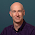Done. Thanks!

10.For the M&M problem, why should we consider the probability of picking the green one since the question is related to yellow? Why not solve it as (p(prior) * p(picking yellow from 1994)) / p(picking yellow from both 1994 and 1996)?

1.It's true that the question is about the yellow M&M, but the answer depends on which bag is which, and the green M&M provides information about that.

To see why, imagine if the green M&M had been blue. That would tell you for sure which bag was which, and that would affect the answer.

Hope that helps.

2.Yes it helps. Thank you for taking time to answer.

3.Mmm, I really have some problem with the M&Ms one. How do you calculate the likelihoods? Seeing the solution, logically I can relate, but I can't formalize.
Originally, I proceeded calculating two separate conditional probability:
P(94|yellow)=0.2/0.34=0.588
P(96|green)=0.2/0.3=0.666
then I was hoping to "combine" the two. I tried:
P(94|((94|yellow),(96|green)))
without luck. Help ^^'.

4.This should work: that is, you should be able to do an update with the yellow M&M followed by an update with the green M&M, and get the same result.

I am working on a new book called Think Bayes that uses this example in Chapter 1:

http://www.greenteapress.com/thinkbayes/html/thinkbayes002.html#toc9

If you look at the way I presented the solution there, it might help.

5.First of all, thanks for your reply. Thanks for the link, too, even if it didn't help with this specific problem (being based on the same material you used for your lecture at the PyCon this year, which I have already checked).
To solve following my original idea, what helped was "updating", I did what follow:
- H-start -> P(94s|Box1)=P(96s|Box1)=0.5
- H-updated -> P(94s|yellow)=P(94u|Box1)=P(96u|Box2)=0.588
- P(96|green)=0.2*0.588/0.15 = 0.784
or
- P(94|yellow)=0.2*0.666/0.17 = 0.784
Just two more questions:
- where does 4% difference come from?
- how would you express formally hypotesis A and B?

6.Here's how I state the hypotheses in Think Bayes:

A: the yellow M&M is from 1994, which implies that green is from 1996.
B: the yellow M&M is from 1996 and green from 1994.

I don't understand your first question: what 4% difference do you mean?

7.Doing "my way" the posterior turns out to be 78%, against the 74% of the proposed solution.

8.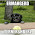Hi Allen, for A: why wouldn't yellow from 1994 be 20/34? 20 in 1994/total yellows between the two. and for B: 14/34?

Is it because we aren't considering the evidence yet?

11.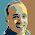Allen, am I missing something with the Elvis question? If 1.9% are twins and .2% are identical, wouldn't it be 2/19 or 10.5% of all twins are identical?

1.Hi David. Odd, isn't it? I suspect that the three numbers in the Wikipedia quote come from different sources, because they are not quite consistent with each other. But since 0.2% is reported with only one significant digit, the result of your division (10.5%) has only one sig fig as well. And at that level of precision, 10.5 and 8 are equal.

2.Thanks Allen, I was probably over thinking it ;) I couldn't find your quote from the wikipedia article (It currently has 1.1%) and was just curious if something was lost in translation.

12.The calculated results from the blood problem can't be right, right (type O and type AB)? For hypothesis X,don't we have to multiply by 2, because the AB perp could be either of the two people at the scene?

1.I think it's correct as written. If Oliver accounts for the type O sample, then there was only one other person at the scene who left a sample, and only one sample to explain, so no factor of two required.

2.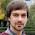Solving this problem was a really cool experience. I first did not accept the idea that evidence consistent with a hypothesis can make the hypothesis less likely and agreed with gary that we need to multiply by 2 in P(E/X). Just because it lead to the intuitive conclusion that the evidence increases the probability that Oliver was one of the two people. However, I then imagined what if Oliver was AB and not 0. Multiplying by 2 would then lead to the impossible probability of 1.2. That is 2*1*0.6 instead of 2*1*0.01.

Only then it came to me that just one sample of 0 really is a little too few should we take Oliver for granted. It was definitely worth being puzzled for a while.

Just a minor technical issue: Since the Bayes's theorem is P(X/E) = P(X)*P(E/X) / P(E)
shouldn't the number 0.012 be labelled P(E) rather than P(E/Y)? Not that it makes much difference here, but it leads to some confusion as of where to plug the number in the theorem. Also imagine the same problem for a population of only say 10 people. In such a situation, P(E) would not be equal to P(E/Y) and I think what we really are interested in is P(E).

3.Jaromir, thanks for your comments -- I'm glad this problem was worth the effort!

I didn't actually apply Bayes's theorem in my solution; I only computed the likelihoods P(E|X) and P(E|Y). The ratio of these likelihoods is the Bayes factor, K, which indicates whether the evidence favors X or Y, and how strong it is.

13.I'm really frustrated with math teachers being universally suck. This page is no exception. Why can't anyone explain how the hell they get their answers? Is it that hard?

Argh.

1.I'm sorry this page didn't work for you. You might want to try Think Bayes (at thinkbayes.com) which presents some of these examples in more detail.

14.Allen, I love this blog post! Thank you for putting it together.

My girlfriend and I have worked through problem 4 together and got to the same answer. In discussing how we would explain this evidence to a jury, we considered the explanation that it is "20% less likely to expect someone of Oliver's blood type at the scene given the evidence." Would you say this is accurate?

We get this by comparing the probabilities 0.01 vs 0.012.

Thanks again!
-Michael

15.I think it would be very hard to explain this result to a jury. Qualitatively, you could say "the evidence would be less likely if Oliver were guilty, so in light of the evidence it is less likely that Oliver is guilty."

To make that quantitative, you could say that the likelihood ratio is 5:6. So if your odds before hearing the evidence were 1:1, your odds after hearing the evidence should be 5:6, or 45%.

But that's probably too much math for a jury.

1.Great point, and thanks for the clarification.

While solving this problem we also calculated the probability that at least 1 person of type O blood be at the scene of the crime and came out to roughly 83% if I recall correctly.

Explaining to the jury that there's an 80%+ chance of a type O at the scene makes it pretty difficult to act on the evidence. If the suspect was non-O blood type it might be a very different story!

Thanks again for the post & the explanation. We really enjoyed working through these practice problems.

-Michael

16.This comment has been removed by the author.

17.Thanks for a great column. The problems illustrate interesting, real-world applications of Bayes Theorem. I would like to say, however, that I believe that your answer to the Monty Hall problem (#6) is not correct. If we are assuming that, after the contestant has made his/her choice, the host will always open the door which does not have the car, then p(A|E) is 1/3 and not 1/2. Therefore, it behooves the contestant to switch doors; it will in fact double his/her chances.

1.Hi and thanks for this comment. In the version of Monty Hall I present here, if the car is behind door A, Monty chooses B with probability p and C with probability 1-p. This is different from the usual statement of the problem, but when p=1/2 it reduces to the usual version with p(A|E)=1/3, as you say.

2.I think what chokurdak khem is pointing out is that your math isn't wrong, your interpretation of the math is wrong. Either that, or I've misunderstood what the problem is.

Consider the following simple python code which gives a monte-carlo solution with p=1. Once you look at that, you'll see that the "full result" is independent of p:

import numpy as np
trials = 100000
(winsbyswitch, winsbynotswitch) = (0, 0)
for door in np.random.randint(1,4,trials):
if door==2: # monte opens door 3 and switching wins
winsbyswitch=winsbyswitch+1
if door==1: # monte opens door 2 and not switching wins
winsbynotswitch=winsbynotswitch+1
if door==3: # monte opens door 2 and switching wins
winsbyswitch=winsbyswitch+1
print "Wins by switch: ",float(winsbyswitch)/float(trials)
print "Wins by notswitch: ",float(winsbynotswitch)/float(trials)

3.Apologies about not being able to figure out how to get the whitespace right in the above post. Also apologies that I didn't make it clear that door==1 is A, door==2 is B, etc.

In the end there are two comments:
1. If the "full problem" is the game where I choose door A and Monty chooses B if possible (i.e. there isn't a goat there) and C otherwise (p=1 case), the answer is that switching still wins 2/3rds of the time. Short answer is that my original choice is right only 1/3rd of the time, and the switching strategy is successful 2/3rds of the time.
2. My comment about "wrong interpretation" is that I think your calculation is correct at calculating the probability that the car is behind door A if Monty opens door B (and it's not there). Which is not the "full problem."

Am I missing something?

4.Hi Ken, You are right, I did not answer the full problem. There are three steps: (1) what is P(A|E)? (2) what should you do? (3) assuming you do the right thing, what is your chance of winning? I only solved (1) and left the rest to the reader. As you said, the answer to (2) is that it is to your advantage to switch, except when p=1 (in which case it doesn't matter). But the answer to (3) depends on p. For example, when p=0 and Monty opens door B, switching to C wins 100% of the time.

5.Thanks!

I was mainly trying to point out what might be some common confusion. Specifically the strategy of "Choosing Door A and Switching" will win 2/3rds of the time. This is what seems contrary to your statement of the solution. Specifically, it does not matter what Monty Hall does.

i.e. Assuming that the car is randomly behind door A,B, or C:

The strategy:
1. Pick Door A.
2. Whatever Monty Hall shows, change from Door A to the remaining door.

The above strategy will win 2/3rds of the time and is _independent_ of p. i.e. Monty Hall could have p=1 and always open Door B if there isn't a car behind. The code (poorly indented as it is) shows why. It underscores that the original choice (Door A) is wrong 2/3rds of the time and, thus, switching is necessarily right 2/3rds of the time and this is independent of Monty Hall's choice of doors (as long has he doesn't open the door with a car ...).

6.Ah, yes. I think you have identified the point of confusion. Your analysis is correct before Monty opens a door. But after Monty opens a door you have more information, and the question asks specifically about the case where Monty opens door B. In that case, your chance of winning is 2/3 only if p=1/2. For other values of p, your chance of winning might be as low as 1/2 or as high as 1.

7.I'm pretty sure that is not correct. Consider the python code I posted in my first post. This simulates draws from the game:

1. Car is randomly put behind door 1, 2, or 3.
2. I pick door 1
3. Monty Hall operates with p=1. i.e. He picks door 2 if he can (it doesn't have a car). Otherwise Monty picks door 3.
4. I switch from door 1 to the door that Monty hall didn't open.

The result is I win 2/3rds of the time.

The probability you calculated (with the p=1 case), is the probability that I win by switching if Monte hall opens Door B (and it's not there) [50%].

8.Good, we are agreeing now. As you say, the probability I report is for the case where Monty opens Door B, because that's what the question asks.

9.Yes. And thanks for your patience!

I think we are on the same page. And (to hopefully further clarify) the "full solution" in the case that Monty Hall behaves according to p=1 is:

P( I win by switching | Monty Hall opens B) * P( Monty Hall opens B) + P( I win by switching | Monty Hall opens C) * P( Monty Hall opens C) = 1/2 * 2/3 + 1 * 1/3 = 2/3

... and you've done the general p case of the first term, i.e. you've calculated P( I win by switching | Monty Hall Opens B)

Thanks!!!

18.Hi, I was looking at and working through these problems.
In problem 6, in the case you described where Monty opens door C, I believe that P(A|E) is (1-p)/(2-p), not (1-p)/(1+p).
This is because I found:
P(E) = 1/3 + (1-p)*1/3
so P(A|E) is (1-p)*1/3 / (1/3 + (1-p)*1/3)
which simplifies to (1-p) / (2-p).

1.You are correct! I will make that correction in the article.

19.Hi,

I would like to inquire about the working for Q2 quoted below:

"The likelihoods are
P(E|A) = (0.2)(0.2)
P(E|B) = (0.1)(0.14)

So P(A|E) = 40 / 54 ~ 0.74"

Why is P(E|A)= 0.2*0.2 instead of 0.2*0.5?
Why is P(E|B) = 0.1*0.14 instead of 0.5*0.14?

I do not understand since P(A)=P(B) = 0.5

Thanks! (:

1.I added some explanatory text to the article. Please let me know if it answers your question.

20.This comment has been removed by a blog administrator.

21.Hi, for the Elvis' twin problem:

If the percentage of twins in the world pop. is:
#T/#Pop = 1.9%,
and that the percentage of monozygotic twins over the worl pop. is:
#MZT/#Pop = 0.2%

Therefore the percentage of MZT over the number of all twins is:
#MZT/#T= #MZT/#Pop*(#Pop/#MZT) = 0.2/1.9 = 0.105 = 10.5%

Am I wrong ?

1.Odd, isn't it? I suspect that the three numbers in the Wikipedia quote come from different sources, because they are not quite consistent with each other. But since 0.2% is reported with only one significant digit, the result of your division (10.5%) has only one sig fig as well. And at that level of precision, 10.5 and 8 are equal.

22.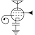OMG, where do I get one of those neon signs? I have never wanted to own a neon sign before. Did you have it made up special?

I have been looking over the "Think Bayes" PDF and fondly remembering the estimation and detection course that I took once upon a time at MIT. THANK YOU for writing the missing textbook for that course.

1.Thanks for your kind words.

Sadly, I don't own that sign. According to this thread:

It lives in the office of Autonomy Corp. The photo is from Wikipedia. I should have given credit and pointed to this page:

http://en.wikipedia.org/wiki/File:Bayes%27_Theorem_MMB_01.jpg

23.Hi Allen.

In 3), you end up with:
P(A|E) = 8/54 ~ 0.15.

How do you determine that P(E) = 0.54 ?

1.I plugged the previous values into Bayes's theorem:

P(A|E) = P(A) P(E|A) / P(E)

Where the denominator P(E) is

P(A) P(E|A) + P(B) P(E|B)

All clear?

24.Ok I see, total probability..., thanks!

25.With regard to question 4, although the question does not ask for posterior probability, I am attempting
to solve it using the posterior probability approach to see which proposition has higher odd of
finding the criminal.

I define 3 propositions which I think are exclusive and exhaustive with regards to this problem,
A: Criminal1 is Oliver. Criminal2 is other.
B: Criminal1 is other. Criminal2 is Oliver.
C: Criminal1 is other. Criminal2 is other.

I then attempt to solve it using thinkbayes.Suite approach.

def bloodTraceProblem():
'''
Two people have left traces of their own blood at the scene of a crime.

A suspect, Oliver, is tested and found to have type O blood.

The blood groups of the two traces are found to be of type O
(a common type in the local population, having frequency 60%)
and of type AB (a rare type, with frequency 1%).

Do these data (the blood types found at the scene) give evidence
in favour of the proposition that Oliver was one of
the two people whose blood was found at the scene?

We define 3 propositions,
A: Criminal1 is Oliver. Criminal2 is other.
B: Criminal1 is other. Criminal2 is Oliver.
C: Criminal1 is other. Criminal2 is other.

Data is the blood trace found at the scene

'''

class BloodTrace(thinkbayes.Suite):

def Likelihood(self, data, hypo):
blood_trace = data

if (hypo == 'A'):
if (blood_trace == "O"):
return 1
elif (blood_trace == 'AB'):
return 0.01
else:
return (1 - 0.6 - 0.01)
elif (hypo == 'B'):
if (blood_trace == 'O'):
return 1
elif (blood_trace == 'AB'):
return 0.01
else:
return (1 - 0.6 - 0.01)
else: # hypothesis C
if (blood_trace == "O"):
return 0.6
elif (blood_trace == 'AB'):
return 0.01
else:
return (1 - 0.6 - 0.01)

blood_trace = BloodTrace("ABC")
blood_trace.Update('O')
blood_trace.Update('AB')

blood_trace.Print()

The output is
A 0.384615384615
B 0.384615384615
C 0.230769230769

The output is suggesting that we have better odd of solving the crime by assuming that Oliver is one of the criminal and to focus
all effort to find the 'AB' guy. This approach narrows the search to 1% of population rather than 60% of the population. The decision may be
different if we believe that there is dependency between criminal 1 and criminal 2 i.e. finding either one will increase the
odd of finding the other because they probably know each other.

I will be glad to hear from you if there is any areas that I may have overlooked in my solution.

26.It would be beneficial for those of us trying to intuit this if, instead of "Plug in Bayes's theorem" we got a little clearer elaboration. Thanks though.

27.I'm having troubles going through the fourth problem.

Why would p(E|X) be equal to .01

I think you get that result from multiplying .01 (The probability of finding AB type blood) by 1, given that it makes sense to find O type blood if Oliver was actually involved in the crime.

But why didn't you multiply that by a factor of 2? Why wouldn't the permutation factor matter in one case (When testing the hypothesis of Oliver being guilty), but it does in the other?

Thank you! :D

1.If Oliver left blood at the scene, he accounts for the O sample, leaving only one sample to be explained. The chance that one random person has AB blood is 1%.

If Oliver did not leave blood at the scene, there are two samples to be explained. If you draw random pairs from the population, you might get [AB, O] or [O, AB] with equal probability, so there are two ways to generate the evidence.

If that's not clear, the best way to prove it to yourself is to enumerate all possible pairs.

28.Thank you for publishing these problems. I had some trouble figuring out how P(E) was derived in the M&M problem (#2). I asked for help on math overflow and got a good answer.. May be helpful to others who got similarly stuck -> http://math.stackexchange.com/questions/1924904/urn-type-problem-with-bayes-theorem-and-mms-dont-understand-how-probability

1.Thanks for that link. The response from Adriano shows another way to answer the question, but it doesn't use Bayes's theorem. I think the method using Bayes's theorem is easier, but maybe not everyone thinks so.